4. MOVING CHARGES AND MAGNETISM

Q.1. Identify the part of the electromagnetic spectrum which is : (i) Suitable for radar system in air craft nevigation. (ii) adjacent to the low frequency end of the electromagnetic spectrum. (iii) produced in nuclear reaction (iv) produced by bombarding a metal target by high speed electrons.

Ans ⇒ (i) Microwave (ii) Radio wave (iii) Gamma rays (iv) X-rays.

Q.2. A cell of e.m.f. (E) and internal resistance (r) is connected across a variable external resistance (R) plot graphs to show the variation of (i) E with R (1) Terminal p.d. of the cell (V) with R.

Ans ⇒ (i) E is treated as constant.
(ii) Increase in R reduces current drawn from the cell. Low R will draw more current from source thereby reducing the terminal voltage V. Large value of R will draw less current from cell so the terminal voltage will increase.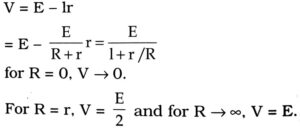Q.3. State the principle and working of a cyclotron. Write two uses of this machine.

Ans ⇒ A positive ion can be accelerated to a very high energy by making it to pass through a moderate electric field again and again by making use of a magnetic field.
It is used to implant ions into solid and modify their properties. It is also used in hospitals to produce radioactive substance.

Q.4. Give the formula for the magnetic field produced by a straight infinitely long current carrying wire. Describe the lines of field in this case.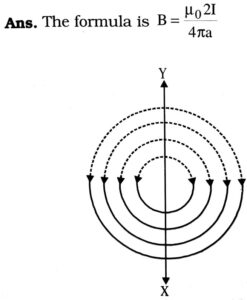The lines of magnetic field due to long straight conductor are concentric circles with the conductor as the centre, lying in plane perpendicular to the straight conductor along clockwise if current is flowing from X to Y as shown in Figure.

Q.5. What is the magnetic effect of current ? Describe the nature of the magnetic field related with the nature of current.

Ans ⇒ When a current is passed through a onductor, magnetic field is produced around the
iductor. It is called magnetic effect of current. The Monetic field is in the form of concentric magnetic lines of force for a linear conductor carrying current. The magnetic field is in the form of parallel straight lines at the centre and concentric magnetic lines the circular coil carrying current.

Q.6. A particle of mass ‘m’ with charge ‘q’ moving with a uniform speed ‘v’ normal to a uniform magnetic field ‘B’ describes a circular path of radius r. Derive expressions for the (i) time period of revolution and (ii) kinetic energy of the particle.

Ans ⇒ (i) Centripetal force the charged ion = Force due to perpendicular magnetic field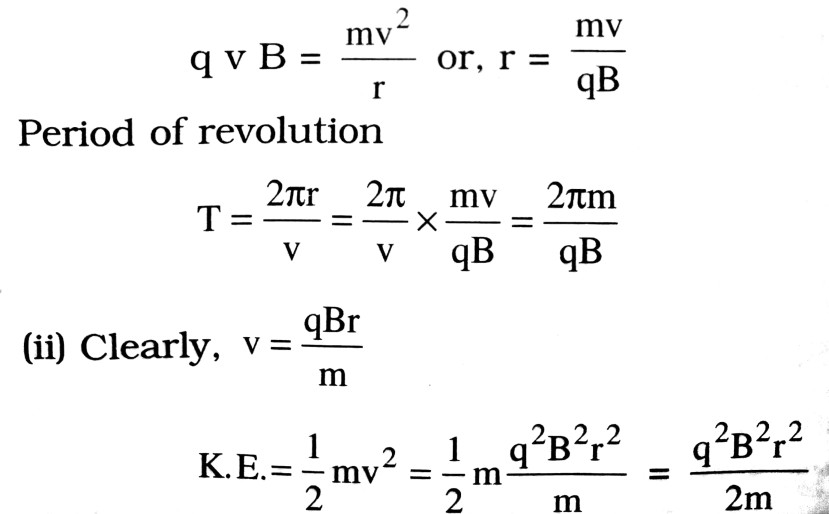Q.7. In what respect does a wire carrying a current differ from a wire, which carries no current ?

Ans ⇒ A current carrying wire produces a magnetic field. When current is flowing through a wire the electrons move inside it along a definite direction. On the other hand, if no current is flowing through a wire, the electrons inside this wire are in random motion, their average thermal velocity is zero. Such a wire does not produce any magnetic field.

Q.8. In what ways electric and magnetic field are different ?

Ans ⇒ (i) Electric field is due to charges at rest as well as in motion, whereas magnetic field is due to magnet or current through a conductor.

(ii) The strength of electric field at a point decreases with the dielectric medium but the strength of magnetic field increases when a permeable medium is inserted there.

(iii) The electric lines of form representing the electric field do not form a closed path, whereas the magnetic lines of force form a closed path.

Q.9. How does (i) an ammeter (ii) voltmeter differ from a galvanometer ?

Ans ⇒ (i) Ammeter is a low resistance galvanometer Men a low resistance shunt is connected across galvanometer, it becomes ammeter.

(ii) Voltmeter is a high resistance galvanometer. When a suitable resistance is connected in series of galvanometer, it becomes voltmeter.

Q. 10. Two parallel wires carrying current in the same direction attract each other while two beams of electrons travelling in the same direction repel each other. Why ?

Ans ⇒ Two parallel wires carrying currents in the same direction attract each other due to magnetic interaction between two wires carrying currents because the current in a wire produces a magnetic field and the magnetic interaction is of attractive nature when current in the two parallel wires in the same direction. The two beams of electrons travelling in the same direction will be a source of both an electric and magnetic field. Due to magnetic interaction there will be force of attraction between the two moving electrons but due to electrostatic interaction, there will be a force of repulsion between them. If the beams of electrons are moving slowly, the electrostatic force of repulsion between them. If the beams of electrons are moving slowly, the electrostatic force of repulsion between the electrons dominates the magnetic attraction between them.

Q.11. (a) State Ampere’s Law connecting the line integral of B over a closed path to the net current crossing the area bounded by the path.

(b) Use Ampere’s Law to derive the formula for magnetic field due to an infinitely long straight current-carrying wire.

(c) Explain carefully why the some derivation as in (b) is not valid for magnetic field in a plane normal to a current-carrying straight wire of finite length and passing through the mid-point of the wire.

Ans ⇒ (a) Ampere circuital law states that the line integral of magnetic field B round any closed path is μ0 times the current threading the closed path mathematically.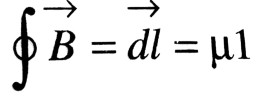(b) To derive Ampere Circuital Law of magnetic field due to an infinite straight conductor, consider a long straight conductor XY such that current I flows through it from X to Y. There will be magnetic field around wire which can be derived as follows :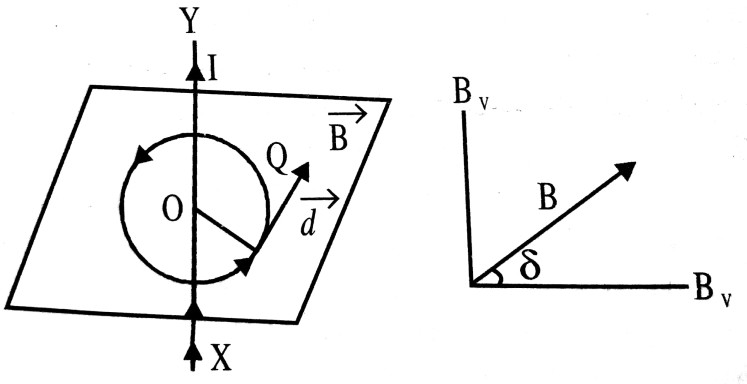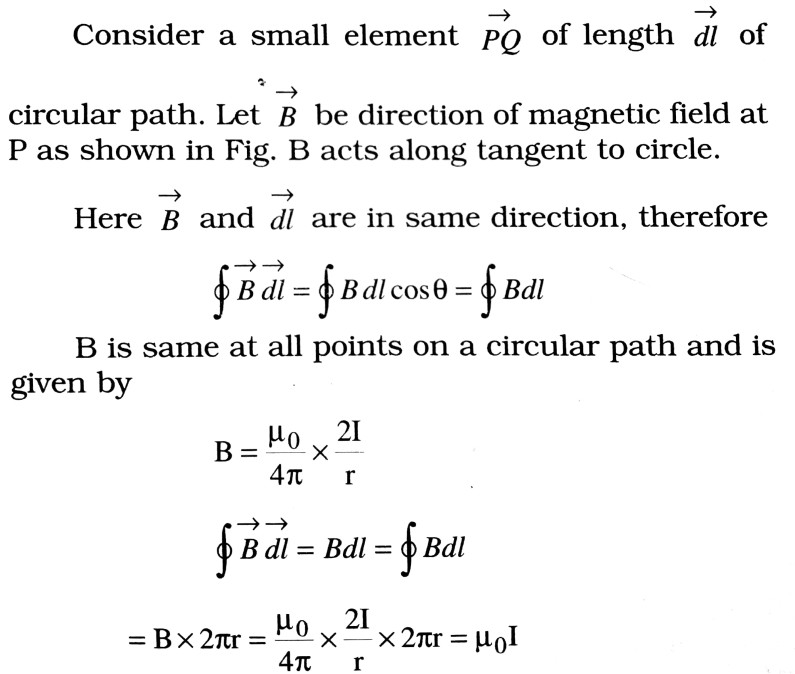Hence Ampere Circuital Law is proved.
(c) A straight conductor of finite length cannot by itself form a complete steady current circuit. Additional conductors are necessary to close the circuit. These will spoil the symmetry of the problem. The difficulty disappears if the conductor is infinitely long.

Q.12. A cyclotron is not suitable to accelerate electrons Why ?

Ans ⇒ When an electron is accelerated in a cyclotron very soon it acquires a very high velocity. Due to which its mass increases with velocity according to relation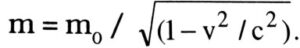Therefore the time taken by electron to describe semicircular path inside the dee of a cyclotron, t = m /Bq. also increases with the increase of m. Due to which the electron does not arrive in a gap between the two dees exactly at the instant when the polarity of the two dees changes. Due to which the electron goes out of step with the oscillating electric field and hence cannot be accelerated by cyclotron.

Q.13. Why should a voltmeter have a high resistance and a low current carrying capacity ?

Ans ⇒ Voltmeter is a high resistance galvanometer. It is used to measure potential difference between two points of the circuit. To measure a potential difference between two points of a circuit, the voltmeter is connected in parallel to the circuit across those two points. The potential differences those two points will not be affected if there is no change in the current flowing through the circuit between those two points, which will be so if practically no current flows through voltmeter. The same is possible if voltmeter has a high resistance and a low current carrying capacity. That is why a voltmeter should have high resistance and low current capacity.

Q.14. Why should an ammeter have a low resistance and a high current carrying capacity ?

Ans ⇒ An ammeter is used to measure the current. It can measure the current of the circuit if connected in series of the circuit. The ammeter in series of the circuit can measure the current and will not disturb the current of the circuit if its resistance is low and current carrying capacity is high.

Q.15. Define self inductance and give its SI unit. Derive an expression for the self inductance of a long solenoid.

Ans ⇒ The self inductance of a coil is the magnetic flux linked with it when unit current flows through it. Its ‘Sl’ unit is Henry.

Self inductance of a long solenoid : Consider a long solenoid carrying a current i.e. Magnetic field at any point inside it is B = μ0 ni
where n is the number of turns per unit length. If A is area of each turn, the magnetic flux linked with each turn of the solenoid is
ϕ = BA = (μ0ni)A
Total magnetic flux linked with the solenoid is
Φ = ϕ x number turns = (μ0ni A) N Self inductance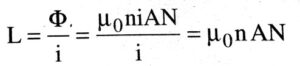Since N = nl, 1 being the length of the solenoid, we have L = μ0n2AL.

Q.16. An electron moving through a field remains undeflected. Is it possible that there is no magnetic field ?

Ans ⇒ In the pressure of a magnetic field also a moving electron may remain undeflected because when the electron moves in parallel or antiparallel magnetic field, no force acts on the electron. Therefore moves without deviation.

Q.17. State Maxwell’s modification of Ampere’s circuital law.

Ans ⇒ As Ampere’s circuital law states that the line integral of magnetic field is the current threading the loop.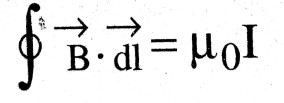Where I is the conduction current. Maxwell modified this statement on the basis of fact that the time varying magnetic field produces time varying electric field and time varying electric field produces the current called displacement current which again produces the time varying magnetic field.                   ∴ The Ampere’s circuital law is modified as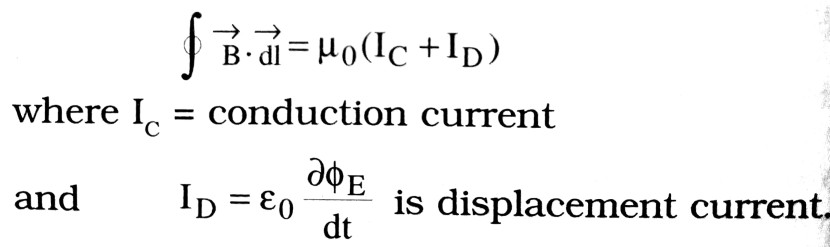## Class 12th physics Subjective question in English

 S.N Physics Short Type Question English Medium 1. ELECTRIC CHARGES AND FIELDS 2. LECTROSTATIC POTENTIAL AND CAPACITANCE 3. CURRENT ELECTRICITY 4. MOVING CHARGES AND MAGNETISM 5. MAGNETISM AND MATTER 6. ELECTROMAGNETIC INDUCTION 7. ALTERNATING CURRENT 8. ELECTROMAGNETIC WAVES 9. RAY OPTICS AND OPTICAL INSTRUMENTS 10. WAVE OPTICS 11. DUAL NATURE OF MATTER AND RADIATION 12. ATOMS 13. NUCLEI 14. SEMI CONDUCTOR ELECTRONICS 15. COMMUNICATION SYSTEMS